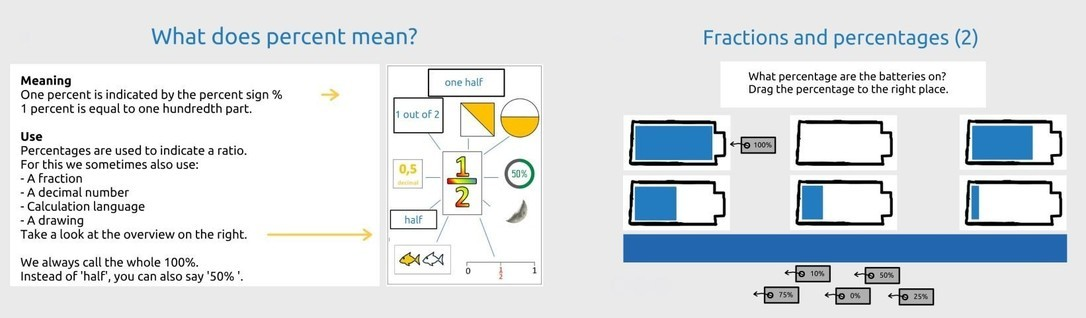Percentages part 2

View this page on a larger screen to send this lesson directly to Presenter

Purpose

• Learn about percentages

This lesson is the second part of the learning line percentages. Therefore, first look back at the lesson contents of part 1. Start the assignments and practice with naming the correct percentages. Calculate percentages in contexts and learn how to convert a percentage into a fraction or decimal number.